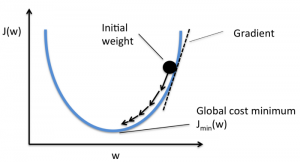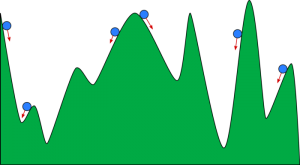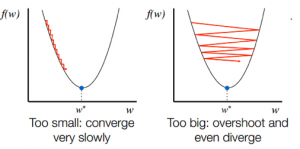HomeBlogArtificial IntelligenceGradient Descent Algorithm: Methodology, Variants & Best Practices

# Gradient Descent Algorithm: Methodology, Variants & Best Practices

Last updated:
12th Jun, 2023
Views
6 MinsView AllOptimization is an integral part of machine learning. Almost all machine learning algorithms have an optimization function as a crucial segment. As the word suggests, optimization in machine learning is finding the optimal solution to a problem statement.

## Best Machine Learning and AI Courses Online

 Master of Science in Machine Learning & AI from LJMU Executive Post Graduate Programme in Machine Learning & AI from IIITB Advanced Certificate Programme in Machine Learning & NLP from IIITB Advanced Certificate Programme in Machine Learning & Deep Learning from IIITB Executive Post Graduate Program in Data Science & Machine Learning from University of Maryland To Explore all our courses, visit our page below. Machine Learning Courses

In this article, you’ll read about one of the most widely used optimization algorithms, gradient descent. The gradient descent algorithm can be used with any machine learning algorithm and is easy to comprehend and implement. So, what exactly is gradient descent? By the end of this article, you’ll have a clearer understanding of the gradient descent algorithm and how it can be used to update the model’s parameters.

## In-demand Machine Learning Skills

 Artificial Intelligence Courses Tableau Courses NLP Courses Deep Learning Courses

Get Machine Learning Certification from the World’s top Universities. Earn Masters, Executive PGP, or Advanced Certificate Programs to fast-track your career.

Before going deep into the gradient descent algorithm, you should know what cost function is. The cost function is a function used to measure the performance of your model for a given dataset. It finds the difference between your predicted value and expected value, thus quantifying the error margin.

The goal is to reduce the cost function so that the model is accurate. To achieve this goal, you need to find the required parameters during the training of your model. Gradient descent is one such optimization algorithm used to find the coefficients of a function to reduce the cost function. The point at which cost function is minimum is known as global minima.Source

Machine learning models and neural networks are frequently trained using the optimization algorithm known as gradient descent. The cost function in gradient descent especially serves as a barometer, measuring the model’s accuracy with each iteration of parameter updates. Training data is used to assist these models learning over time. The model will keep altering its parameters until the function is close to or equal to zero to produce the least inaccuracy. Machine learning models can be effective tools for computer science and artificial intelligence (AI) applications once they are accuracy-optimized.

Before getting into the code, one more concept needs to be defined: what is a gradient? It is intuitively understood to be the slope of a curve at a given position in a particular direction. It is just the first derivative at a particular location in the case of a univariate function.

## The intuition behind the Gradient Descent algorithm

Suppose you have a large bowl similar to something you’ve your fruit in. This bowl is the plot for the cost function. The bottom of the bowl is the best coefficient for which the cost function is minimum. Different values are used as the coefficients to calculate the cost function. This step is repeated until the best coefficients are found.

You can imagine gradient descent as a ball rolling down a valley. The valley is the plot for the cost function here. You want the ball to reach the bottom of the valley, where the bottom of the valley represents the least cost function. Depending on the start position of the ball, it may rest on many bottoms of the valley. However, these bottoms may not be the lowest points and are known as local minima.Source

The calculation of gradient descent begins with the initial values of coefficients for the function being set as 0 or a small random value.

coefficient = 0 (or a small value)

• The cost function is calculated by putting this value of the coefficient in the function.

Cost function = f(coefficient)

• We know from the concept of calculus that the derivative of a function is the slope of the function. Calculating the slope will help you to figure out the direction to move the coefficient values. The direction should be such that you get a lower cost(error) in the next iteration.

del = derivative(cost function)

•  After knowing the direction of downhill from the slope, you update the coefficient values accordingly. A learning rate (alpha) can be selected to control how much these coefficients will change in each iteration. You need to make sure that this learning rate is not too high nor too low.

coefficient = coefficient – (alpha * del)

• This process is repeated until the cost function becomes 0 or very close to 0.

f(coefficient) = 0 (or close to 0)

The selection of the learning rate is important. Selecting a very high learning rate can overshoot the global minima. On the contrary, a very low learning rate can help you reach the global minima, but the convergence is very slow, taking many iterations.Source

## Variants of Gradient Descent Algorithm

Batch gradient descent is one of the most used variants of the gradient descent algorithm. The cost function is computed over the entire training dataset for every iteration. One batch is referred to as one iteration of the algorithm, and this form is known as batch gradient descent.

In some cases, the training set can be very large. In these cases, batch gradient descent will take a long time to compute as one iteration needs a prediction for each instance in the training set. You can use the stochastic gradient descent in these conditions where the dataset is huge. In stochastic gradient descent, the coefficients are updated for each training instance and not at the end of the batch of instances.

Both batch gradient descent and stochastic gradient descent have their pros and cons. However, using a mixture of batch gradient descent and stochastic gradient descent can be useful. In mini-batch gradient descent, neither the entire dataset is used nor do you use a single instance at a time. You take into consideration a group of training examples. The number of examples in this group is lesser than the entire dataset, and this group is known as a mini-batch.

## Popular AI and ML Blogs & Free Courses

 IoT: History, Present & Future Machine Learning Tutorial: Learn ML What is Algorithm? Simple & Easy Robotics Engineer Salary in India : All Roles A Day in the Life of a Machine Learning Engineer: What do they do? What is IoT (Internet of Things) Permutation vs Combination: Difference between Permutation and Combination Top 7 Trends in Artificial Intelligence & Machine Learning Machine Learning with R: Everything You Need to Know AI & ML Free Courses Introduction to NLP Fundamentals of Deep Learning of Neural Networks Linear Regression: Step by Step Guide Artificial Intelligence in the Real World Introduction to Tableau Case Study using Python, SQL and Tableau

## Best Practices for Gradient Descent Algorithm

• Map cost versus time: Plotting the cost with respect to time helps you visualize whether the cost is decreasing or not after each iteration. If you see the cost to remain unchanged, try updating the learning rate.
• Learning rate: The learning rate is very low and is often selected as 0.01 or 0.001. You need to try and see which value works best for you.
• Rescale inputs: The gradient descent algorithm will minimize the cost function faster if all the input variables are rescaled to the same range, such as [0, 1] or [-1, 1].
• Less passes: Usually, the stochastic gradient descent algorithm doesn’t need more than 10 passes to find the best coefficients.

## When To Use Gradient Descent Algorithm?

When parameters need to be found via an optimization technique but cannot be determined analytically (for example, using linear algebra), gradient descent is the method of choice.

• Towards the minimum, a more direct route is adopted.
• Since updates are needed after an epoch has run, computations must be efficient.
• It is simpler to fit into the memory that has been allocated.
• Gradient descent convergence is produced that is stable.

• Can converge at nearby saddle and minima sites.
• Slower learning since an update is only made after we have examined every observation.
• For huge datasets, perform duplicate computations for the same training sample.
• Large datasets might not fit in the memory, making it exceedingly slow and difficult to solve.
• We can update the model’s weights with the fresh data because we compute the full dataset.

## Wrapping up

You get to know the role of gradient descent in optimizing a machine learning algorithm. One important factor to keep in mind is choosing the right learning rate for your gradient descent algorithm for optimal prediction.

upGrad provides a PG Diploma in Machine Learning and AI and a  Master of Science in Machine Learning & AI that may guide you toward building a career. These courses will explain the need for Machine Learning and further steps to gather knowledge in this domain covering varied concepts ranging from gradient descent algorithms to Neural Networks.Blog Author
Director of Engineering @ upGrad. Motivated to leverage technology to solve problems. Seasoned leader for startups and fast moving orgs. Working on solving problems of scale and long term technology strategy.
Get Free Consultation

Select CourseSelectBy clicking 'Submit' you Agree to

#### Our Popular Machine Learning Course

11. What concept underlies the Gradient Descent?

To locate a differentiable function's local minimum, an optimization process known as a gradient descent is used. The basic goal of gradient descent in Machine Learning is to move in the gradient's opposite direction. This will result in the steepest decline and, ultimately, the lowest point.

22. What is the principal drawback of the gradient descent algorithm?

The Gradient descent algorithm has the drawback that the weight update at a given time (t) is solely determined by the learning rate and gradient. The previous steps when navigating the cost space are not considered.

33. What are some popular Gradient Descent methods?

Some popular approaches to gradient descent in Machine Learning are: Batch gradient descent: a method in which the complete training set is considered before moving forward. Convex and largely smooth describes its cost function. Stochastic Gradient Descent: A single decision is made by only considering one piece of data. Its cost function fluctuates, but as more iterations go by, the fluctuations eventually get smaller. Mini-batch Gradient Descent: where a batch of a fixed number of data is considered. Its cost function is also a fluctuation one.

## Suggested Blogs

90917
AWS Projects & Topics Looking for AWS project ideas? Then you’ve come to the right place because, in this article, we’ve shared multiple AWS proj